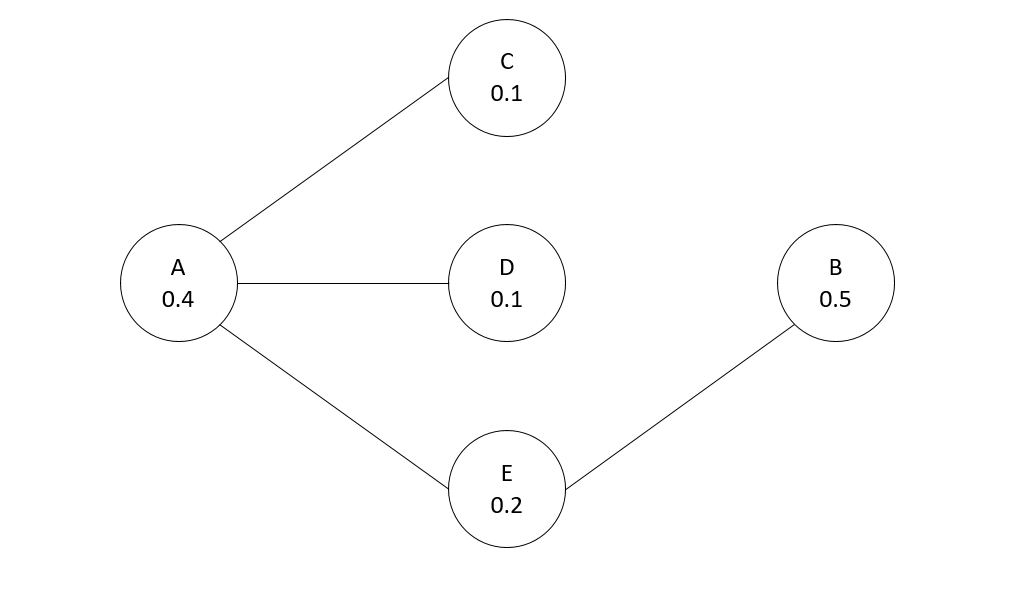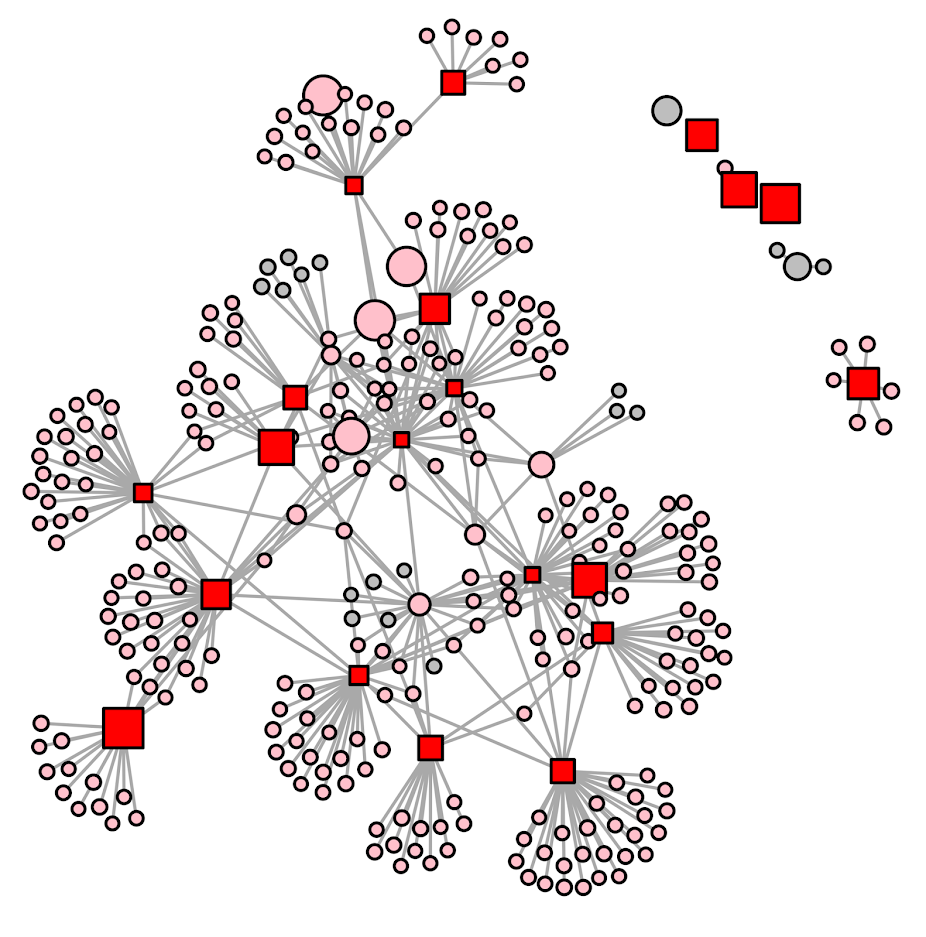# Optimal treatment assignment with network spillovers

Motivated by a recent piece by Wood and Papachristos (2019), (WP from here on) which finds if you treat an individual at high risk for gun shot victimization, they have positive spillover effects on individuals they are connected to. This creates a tricky problem in identifying the best individuals to intervene with given finite resources. This is because you may not want to just choose the people with the highest risk – the best bang for your buck will be folks who are some function of high risk and connected to others with high risk (as well as those in areas of the network not already treated).

For a simplified example consider the network below, with individuals baseline probabilities of future risk noted in the nodes. Lets say the local treatment effect reduces the probability to 0, and the spillover effect reduces the probability by half, and you can only treat 1 node. Who do you treat?We could select the person with the highest baseline probability (B), and the reduced effect ends up being 0.5(B) + 0.1(E) = 0.6 (the 0.1 is for the spillover effect for E). We could choose node A, which is a higher baseline probability and has the most connections, and the reduced effect is 0.4(A) + 0.05(C) + 0.05(D) + 0.1(E) = 0.6. But it ends up in this network the optimal node to choose is E, because the spillovers to A and B justify choosing a lower probability individual, 0.2(E) + 0.2(A) + 0.25(B) = 0.65.

Using this idea of a local effect and a spillover effect, I formulated an integer linear program with the same idea of a local treatment effect and a spillover effect:$\text{Maximize} \{ \sum_{i = 1}^n (L_i\cdot p_{li} + S_i \cdot p_{si}) \}$

Where$p_{li}$ is the reduction in the probability due to the local effect, and$p_{si}$ is the reduction in the probability due to the spillover effect. These probabilities are fixed values you know at the onset, e.g. estimated from some model like in Wheeler, Worden, and Silver (2019) (and Papachristos has related work using the network itself to estimate risk). Each node, i, then gets two decision variables;$L_i$ will equal 1 if that node is treated, and$S_i$ will equal 1 if the node gets a spillover effect (depending on who is treated). Actually the findings in WP show that these effects are not additive (you don’t get extra effects if you are treated and your neighbors are treated, or if you have multiple neighbors treated), and this makes it easier to keep the problem on the probability scale. So we then have our constraints:

1.$L_i , S_i \in \{ 0,1 \}$
2.$\sum L_i = K$
3.$S_i \leq 1 + -1\cdot L_i , \forall \text{ Node}_i$
4.$\sum_{\text{neigh}(i)} L_j \geq S_i , \forall \text{ Node}_i$

Constraint 1 is that these are binary 0/1 decision variables. Constraint 2 is we limit the number of people treated to K (a value that we choose). Constraint 3 ensures that if a local decision variable is set to 1, then the spillover variable has to be set to 0. If the local is 0, it can be either 0 or 1. Constraint 4 looks at the neighbor relations. For Node i, if any of its neighbors local treated decision variable is set to 1, the Spillover decision variable can be set to 1.

So in the end, if the number of nodes is n, we have 2*n decision variables and 2*n + 1 constraints, I find it easier just to look at code sometimes, so here is this simple network and problem formulated in python using networkx and pulp. (Here is a full file of the code and data used in this post.)

####################################################
import pulp
import networkx

Nodes = ['a','b','c','d','e']
Edges = [('a','c'),
('a','d'),
('a','e'),
('b','e')]

p_l = {'a': 0.4, 'b': 0.5, 'c': 0.1, 'd': 0.1,'e': 0.2}
p_s = {'a': 0.2, 'b': 0.25, 'c': 0.05, 'd': 0.05,'e': 0.1}
K = 1

G = networkx.Graph()

P = pulp.LpProblem("Choosing Network Intervention", pulp.LpMaximize)
L = pulp.LpVariable.dicts("Treated Units", [i for i in Nodes], lowBound=0, upBound=1, cat=pulp.LpInteger)
S = pulp.LpVariable.dicts("Spillover Units", [i for i in Nodes], lowBound=0, upBound=1, cat=pulp.LpInteger)

P += pulp.lpSum( p_l[i]*L[i] + p_s[i]*S[i] for i in Nodes)
P += pulp.lpSum( L[i] for i in Nodes ) == K

for i in Nodes:
P += pulp.lpSum( S[i] ) = S[i]

P.solve()

#Should select e for local, and a & b for spillover
print(pulp.value(P.objective))
print(pulp.LpStatus[P.status])

for n in Nodes:
print([n,L[n].varValue,S[n].varValue])
####################################################

And this returns the correct results, that node E is chosen in this example, and A and B have the spillover effects. In the linked code I provided a nicer function to just pipe in your network, your two probability reduction estimates, and the number of treated units, and it will pipe out the results for you.

For an example with a larger network for just proof of concept, I conducted the same analysis, choosing 20 people to treat in a network of 311 nodes I pulled from Rostami and Mondani (2015). I simulated some baseline probabilities to pipe in, and made it so the local treatment effect was a 50% reduction in the probability, and a spillover effect was a 20% reduction. Here red squares are treated, pink circles are the spill-over, and non-treated are grey circles. It did not always choose the locally highest probability (largest nodes), but did tend to choose highly connected folks also with a high probability (but also chose some isolate nodes with a high probability as well).This problem is solved in an instant. And I think out of the box this will work for even large networks of say over 100,000 nodes (I have let CPLEX churn on problems with near half a million decision variables on my desktop overnight). I need to check myself to make 100% sure though. A simple way to make the problem smaller if needed though is to conduct the analysis on subsets of connected components, and then shuffle the results back together.

Looking at the results, it is very similar to my choosing representatives work (Wheeler et al., 2019), and I think you could get similar results with just piping in 1’s for each of the local and spillover probabilities. One of the things I want to work on going forward though is treatment non-compliance. So if we are talking about giving some of these folks social services, they don’t always take up your offer (this is a problem in choose rep’s for call ins as well). WP actually relied on this to draw control nodes in their analysis. I thought for a bit the problem with treatment non-compliance in this setting was intractable, but another paper on a totally different topic (Bogle et al., 2019) has given me some recent hope that it can be solved.

This same idea is also is related to hot spots policing (think spatial diffusion of benefits). And I have some ideas about that to work on in the future as well (e.g. how wide of net to cast when doing hot spots interventions given geographical constraints).

• Bogle, J., Bhatia, N., Ghobadi, M., Menache, I., Bjørner, N., Valadarsky, A., & Schapira, M. (2019). TEAVAR: striking the right utilization-availability balance in WAN traffic engineering. In Proceedings of the ACM Special Interest Group on Data Communication (pp. 29-43).
• Rostami, A., & Mondani, H. (2015). The complexity of crime network data: A case study of its consequences for crime control and the study of networks. PloS ONE, 10(3), e0119309.
• Wheeler, A. P., McLean, S. J., Becker, K. J., & Worden, R. E. (2019). Choosing Representatives to Deliver the Message in a Group Violence Intervention. Justice Evaluation Journal, Online First.
• Wheeler, A. P., Worden, R. E., & Silver, J. R. (2019). The Accuracy of the Violent Offender Identification Directive Tool to Predict Future Gun Violence. Criminal Justice and Behavior, 46(5), 770-788.
• Wood, G., & Papachristos, A. V. (2019). Reducing gunshot victimization in high-risk social networks through direct and spillover effects. Nature Human Behaviour, 1-7.

# Finding the dominant set in a network (python)

My paper, Choosing representatives to deliver the message in a group violence intervention, is now published online at the Justice Evaluation Journal. For those who don’t have access to that journal, here is a link good for 50 e-prints (for a limited time), and here is a pre-print version, and you can always send me an email for the published copy.

I’ve posted Python code to replicate the analysis, including the original network nodes and edges group data. I figured I would go through a quick example of applying the code for others to use the algorithm.

The main idea is that for a focused deterrence initiative, for the call-ins you want to identify folks to spread the deterrence message around the network. When working with several PDs I figured looking at who was called in would be interesting. Literally the first network graph I drew was below on the left — folks who were called in are the big red squares. This was one of the main problem gangs, and the PD had done several call-ins for over a year at this point. Those are not quite the worst set of four folks to call-in based on the topology of the network, but damn close.But to criticize the PD I need to come up with a better solution — which is the graph on the right hand side. The larger red squares are my suggested call-ins, and they reach everyone within one step. That means everyone is at most just one link away from someone who attended the call-in. This is called a dominant set of a graph when all of the graph is colored in.

Below I give a quicker example using my code for others to generate the dominant set (instead of going through all of the replication analysis). If you are a PD interested in applying this for your focused deterrence initiative let me know!

So first to set up your python code, I import all of the needed libraries (only non-standard is networkx). Then I import my set of functions, named MyFunctions.py, and then change the working directory.

############################################################
#The libraries I need

import itertools
import networkx as nx
import csv
import sys
import os

#Now importing my own functions I made
locDir = r'C:\Users\axw161530\Dropbox\Documents\BLOG\DominantSet_Python'
sys.path.append(locDir)
from MyFunctions import *

#setting the working directory to this location
os.chdir(locDir)
#print(os.getcwd())
############################################################

The next part I read in the CSV data for City 4 Gang 1, both the nodes and the edges. Then I create a networkx graph simply based on the edges. Technically I do not use the node information at all for this, just the edges that list a source and a target.

############################################################
#Reading in the csv files that have the nodes and the edges
#And turning into a networkX graph

#simple function to read in csv files
tup = []
with open(loc) as f:
for row in z:
tup.append(tuple(row))
return tup

#Turning my csv files into networkx objects

#Turning my csv files into networkx objects
C1G4 = nx.Graph()
############################################################

Now it is quite simple, to get my suggested dominant set it is simple as this function call:

ds_C1G4 = domSet_Whe(C1G4)
print(ds_C1G4)

In my current session this gives the edges ['21', '18', '17', '16', '3', '22', '20', '6']. Which if you look to my original graph is somewhat different, but all are essentially single swaps where the best node to choose is arbitrary.

I have a bunch of other functions in the analysis, one of interest will be given who is under probation/parole who are the best people to call in (see the domSet_WheSub function). Again if you are interested in pursuing this further always feel free to reach out to me.